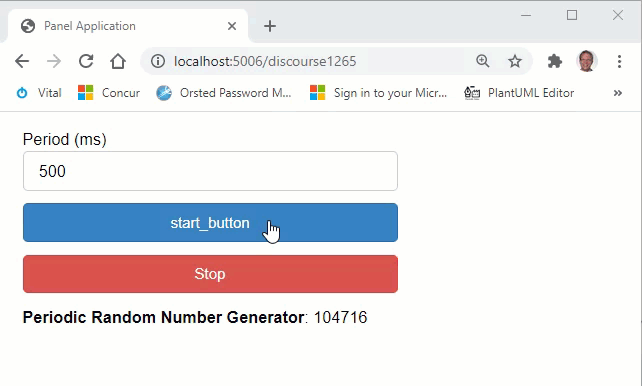# How to interrupt with Events and how to use __main__ with serve

Hi,

I am trying to learn how to make panel useful to me. Note, I do not use jupyter notebooks and am not that familiar with them, all work is done in a .py script. The first question is how do I have “events” in panel get updated by the external browser gui. (An example of what I want to do is below).

The second question is the script only works if the standard name == “main” is commented out when I run bokeh serve myfile.py . Is that by design?

def do_things(event,ar=array):

``````    for element in (ar):

if stop_button.value  == True:
print('Stopped!')
stop_button.value = False
break

#Do some things
print('Still running')
``````

#%%

# if name == “main”:

css = ‘’’
.panel-widget-box {
background: #f0f0f0;
border: 1px black solid;
}
‘’’
array = np.linspace(0,100,1000)
pn.extension(raw_css=[css])

start_button = pn.widgets.Button(name=‘Start’, margin=(5, 5, 5, 5),button_type=‘primary’)
start_button.on_click(do_things)

stop_button = pn.widgets.Toggle(name=‘Stop’, margin=(5, 5, 5, 5),button_type=‘danger’)

col = pn.Column(start_button,stop_button,css_classes=[‘panel-widget-box’])

col.show()

HI @pyquest

Welcome to the community.

Regarding the `name` question you should be using `if name == "__main__":` instead of `if name=="main":`.

Regarding the `event` question. It is difficult for me to understand exactly what you would like to do. Could you be more specific and provide a minimum reproducible example?

Thanks.

Hi Marc,

ya there is a formatting issue with the name = main statement. What I typed is in fact what you typed even if it displays strangely.

Regarding what I wanted to do. I want a start and stop button for some action. I was able to adapt the random number generator to achieve the goal. The solution is below.

import panel as pn
import numpy as np
pn.extension()

def generate_random_number(event=None):
static_text.value = np.random.randint(low=100000, high=200000)

def toggle_periodic_callback(event):
periodic_cb.start()

def update_period(event):
periodic_cb.period = event.new

def abort(event):
periodic_cb.stop()

static_text = pn.widgets.StaticText(name=‘Periodic Random Number Generator’,
value=‘000000’)

generate_button = pn.widgets.Button(name=‘Generate New Number’)
generate_button.on_click(generate_random_number)

start_button = pn.widgets.Button(name='start_button ',
button_type=‘primary’)

start_button .on_click(toggle_periodic_callback)

abort_button = pn.widgets.Button(name=‘Stop’,
button_type=‘danger’)
abort_button.on_click(abort)

period = pn.widgets.Spinner(name=“Period (ms)”, value=500, step=50, start=50)
period.param.watch(update_period, ‘value’)

generate_random_number, period=period.value, start=False) # period in milliseconds

col = pn.Column( period, start_button ,abort_button, static_text)
col.servable()

1 Like

Nice example. Thanks for sharing it back it really showcase how use can use the `periodic_callback`

I put in the code in a format that can be copied``````import panel as pn
import numpy as np

pn.extension()

def generate_random_number(event=None):
static_text.value = np.random.randint(low=100000, high=200000)

def toggle_periodic_callback(event):
periodic_cb.start()

def update_period(event):
periodic_cb.period = event.new

def abort(event):
periodic_cb.stop()

static_text = pn.widgets.StaticText(name="Periodic Random Number Generator", value="000000")

generate_button = pn.widgets.Button(name="Generate New Number")
generate_button.on_click(generate_random_number)

start_button = pn.widgets.Button(name="start_button ", button_type="primary")

start_button.on_click(toggle_periodic_callback)

abort_button = pn.widgets.Button(name="Stop", button_type="danger")
abort_button.on_click(abort)

period = pn.widgets.Spinner(name="Period (ms)", value=500, step=50, start=50)
period.param.watch(update_period, "value")